# RS Aggarwal Solutions for Class 8 Maths Chapter 6 - Operations on Algebraic Expressions Exercise 6C

RS Aggarwal Solutions for the Exercise 6C of Class 8 Maths Chapter 6, Operations on Algebraic Expressions are available here. This exercise has been solved by BYJU’S team experts with utmost care. This exercise has fourteen questions based on the division of polynomials. RS Aggarwal Solutions helps students to maximise their scores in the examinations, while also providing extensive knowledge about the subject. By practising the RS Solutions for class 8, students will be able to grasp the concepts perfectly.

## Download PDF of RS Aggarwal Solutions for Class 8 Maths Chapter 6 – Operations on Algebraic Expressions Exercise 6C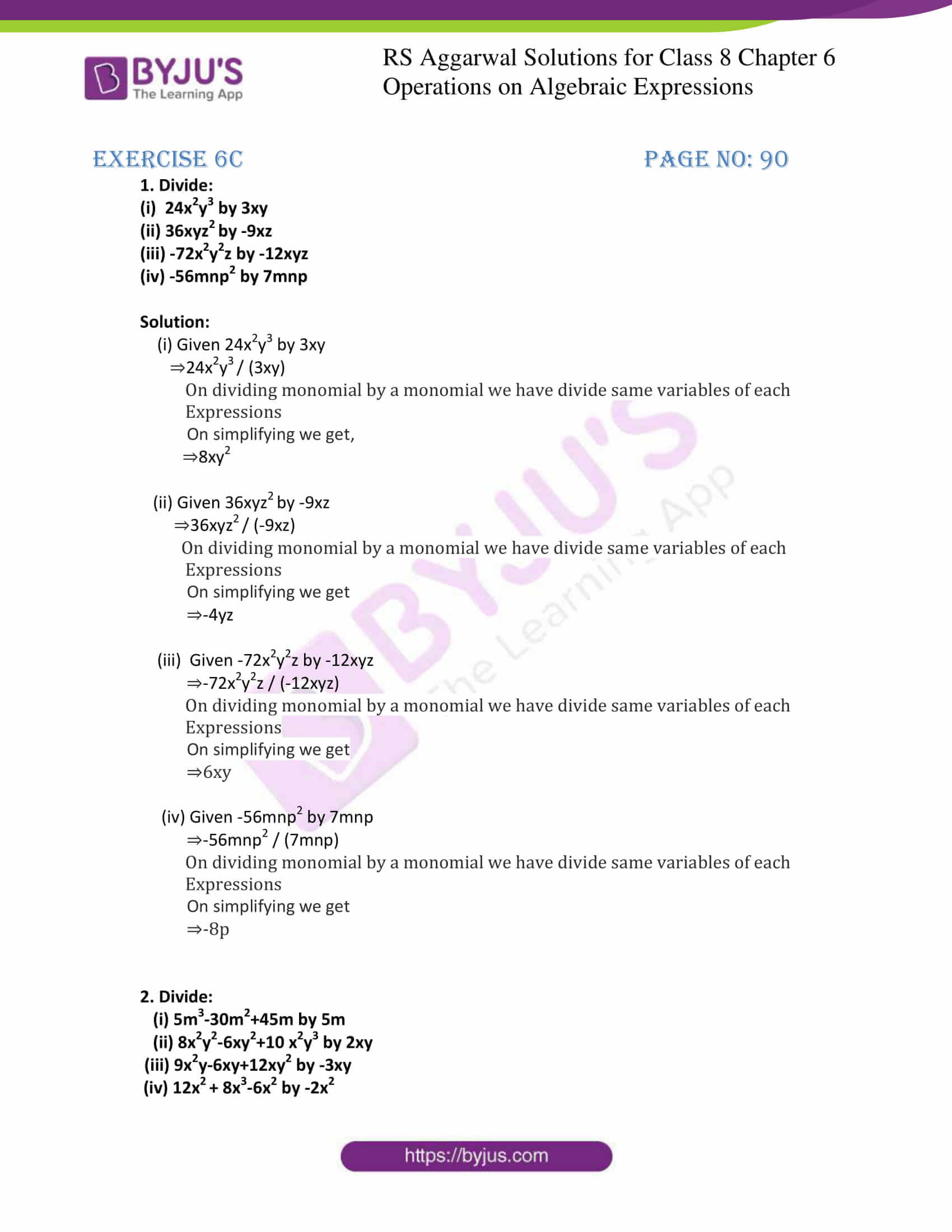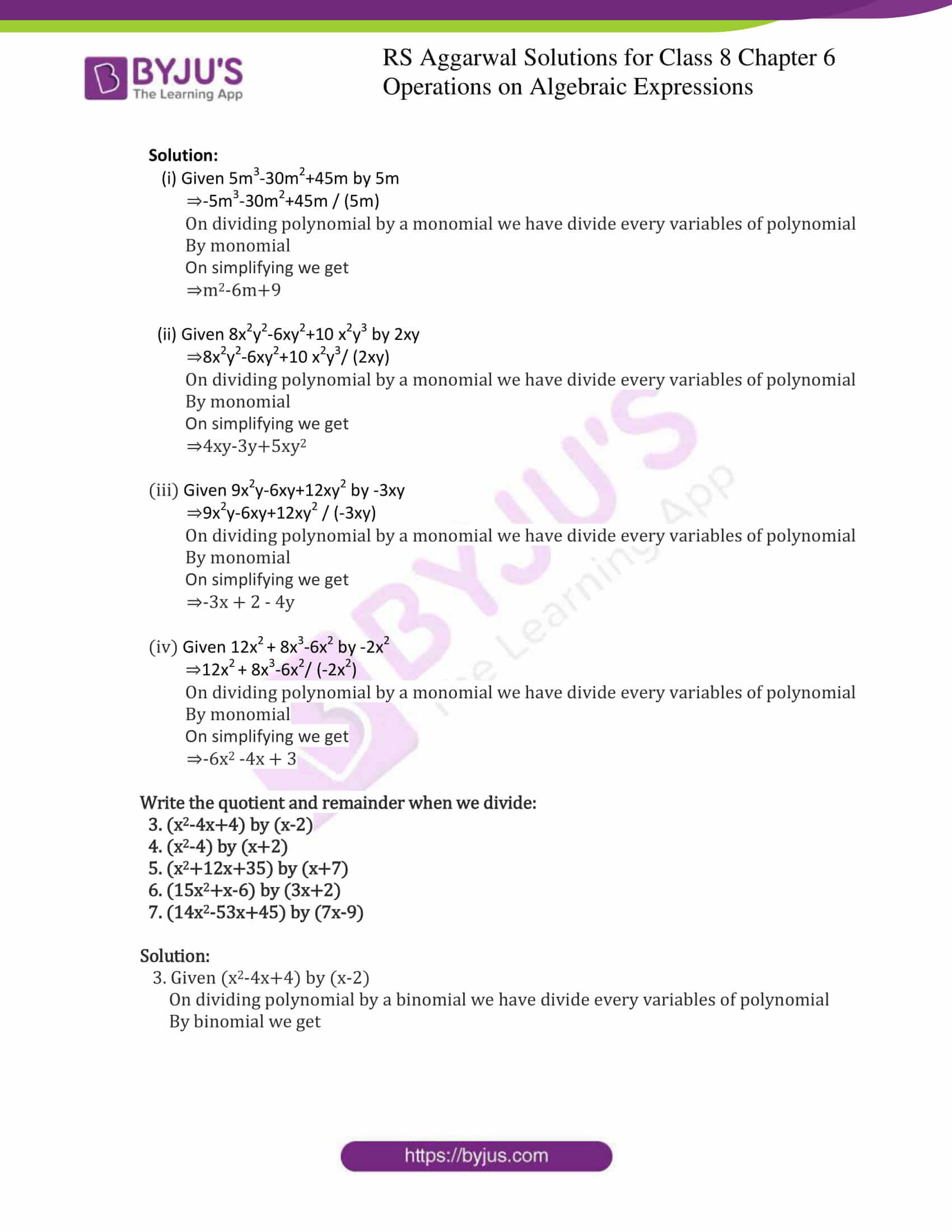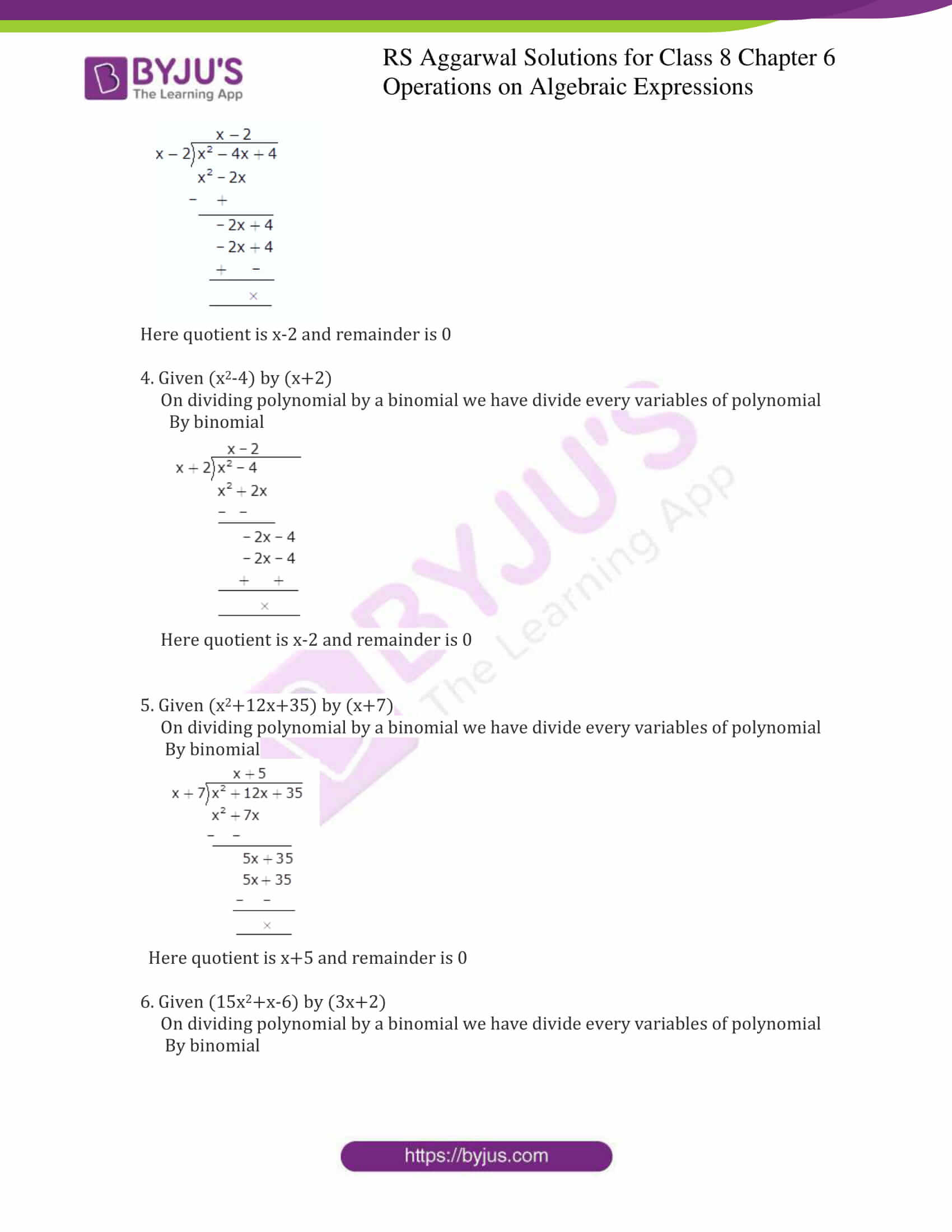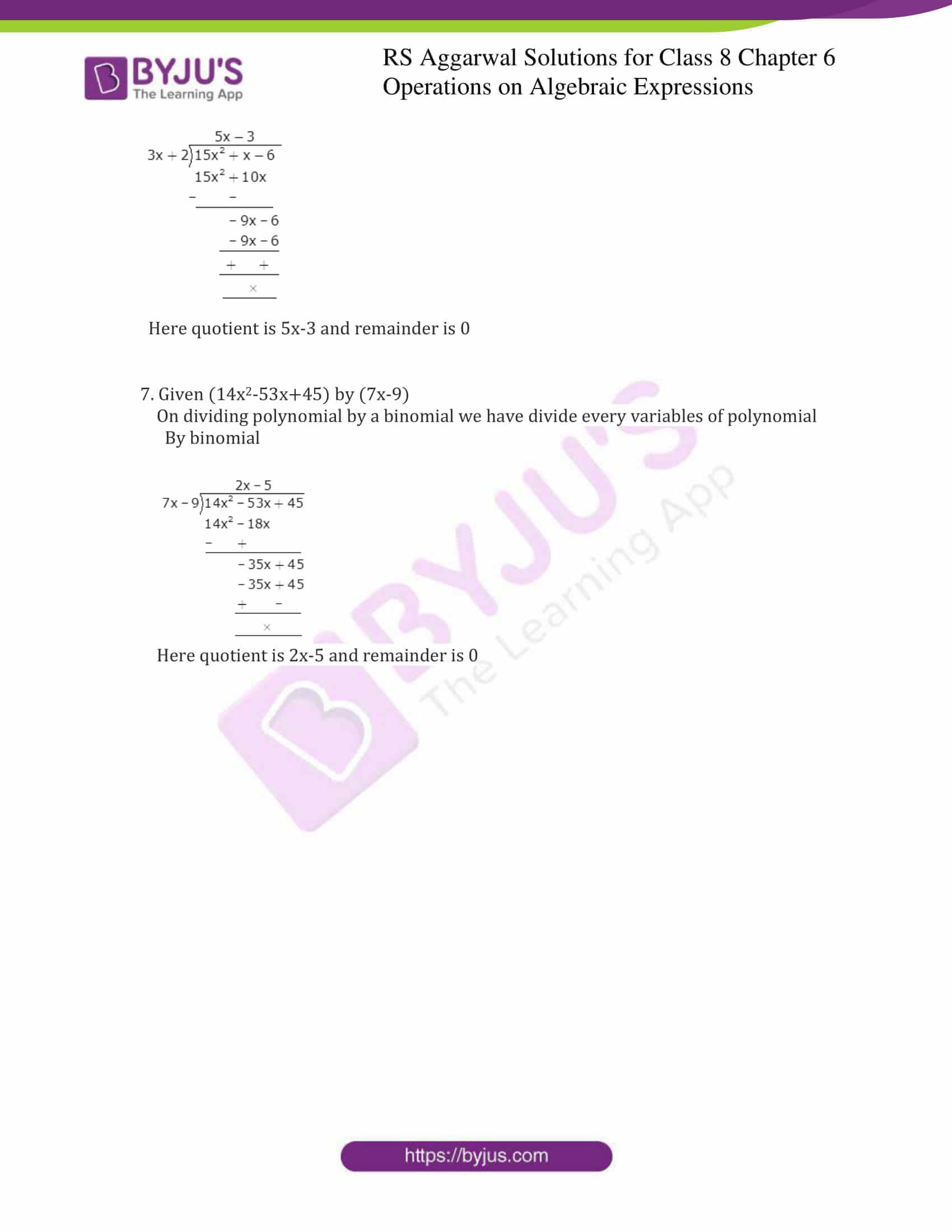### Access answers to Maths RS Aggarwal Solutions for Class 8 Chapter 6 – Operations on Algebraic Expressions Exercise 6C

1. Divide:

(i) 24x2y3 by 3xy

(ii) 36xyz2 by -9xz

(iii) -72x2y2z by -12xyz

(iv) -56mnp2 by 7mnp

Solution:

(i) Given 24x2y3 by 3xy

⇒24x2y3 / (3xy)

On dividing monomial by a monomial we have divide same variables of each

Expressions.

On simplifying we get,

⇒8xy2

(ii) Given 36xyz2 by -9xz

⇒36xyz2 / (-9xz)

On dividing monomial by a monomial we have divide same variables of each

Expressions

On simplifying we get

⇒-4yz

(iii) Given -72x2y2z by -12xyz

⇒-72x2y2z / (-12xyz)

On dividing monomial by a monomial we have divide same variables of each

Expressions

On simplifying we get

⇒6xy

(iv) Given -56mnp2 by 7mnp

⇒-56mnp2 / (7mnp)

On dividing monomial by a monomial we have divide same variables of each

Expressions

On simplifying we get

⇒-8p

2. Divide:

(i) 5m3-30m2+45m by 5m

(ii) 8x2y2-6xy2+10 x2y3 by 2xy

(iii) 9x2y-6xy+12xy2 by -3xy

(iv) 12x2 + 8x3-6x2 by -2x2

Solution:

(i) Given 5m3-30m2+45m by 5m

⇒-5m3-30m2+45m / (5m)

On dividing polynomial by a monomial we have divide every variables of polynomial

By monomial

On simplifying we get

⇒m2-6m+9

(ii) Given 8x2y2-6xy2+10 x2y3 by 2xy

⇒8x2y2-6xy2+10 x2y3/ (2xy)

On dividing polynomial by a monomial we have divide every variables of polynomial

By monomial

On simplifying we get

⇒4xy-3y+5xy2

(iii) Given 9x2y-6xy+12xy2 by -3xy

⇒9x2y-6xy+12xy2 / (-3xy)

On dividing polynomial by a monomial we have divide every variables of polynomial

By monomial

On simplifying we get

⇒-3x + 2 – 4y

(iv) Given 12x2 + 8x3-6x2 by -2x2

⇒12x2 + 8x3-6x2/ (-2x2)

On dividing polynomial by a monomial we have divide every variables of polynomial

By monomial

On simplifying we get

⇒-6x2 -4x + 3

Write the quotient and remainder when we divide:

3. (x2-4x+4) by (x-2)

4. (x2-4) by (x+2)

5. (x2+12x+35) by (x+7)

6. (15x2+x-6) by (3x+2)

7. (14x2-53x+45) by (7x-9)

Solution:

3. Given (x2-4x+4) by (x-2)

On dividing polynomial by a binomial we have divide every variables of polynomial

By binomial we get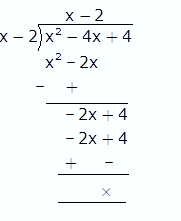Here quotient is x-2 and remainder is 0

4. Given (x2-4) by (x+2)

On dividing polynomial by a binomial we have divide every variables of polynomial

By binomial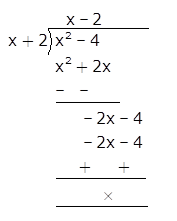Here quotient is x-2 and remainder is 0

5. Given (x2+12x+35) by (x+7)

On dividing polynomial by a binomial we have divide every variables of polynomial

By binomial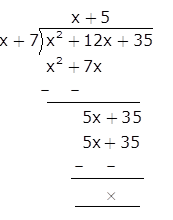Here quotient is x+5 and remainder is 0

6. Given (15x2+x-6) by (3x+2)

On dividing polynomial by a binomial we have divide every variables of polynomial

By binomial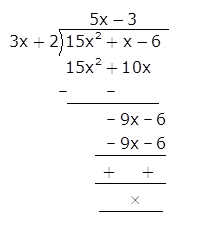Here quotient is 5x-3 and remainder is 0

7. Given (14x2-53x+45) by (7x-9)

On dividing polynomial by a binomial we have divide every variables of polynomial

By binomial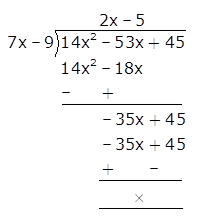Here quotient is 2x-5 and remainder is 0

Exercise 6A

Exercise 6B

Exercise 6D

Exercise 6E

## RS Aggarwal Solutions for Class 8 Maths Chapter 6 – Operations on Algebraic Expressions Exercise 6C

Exercise 6C of RS Aggarwal Solutions for Chapter 6, Operations on Algebraic Expressions deals with the basic concepts related to the division of Algebraic Expressions. Some of the topics focused prior to exercise 6C include the following.

• Division of a monomial by a monomial
• Division of a polynomial by a monomial
• Division of a polynomial by a polynomial

The RS Aggarwal Solutions can help the students in practising and learning each and every concept as it provides solutions to all questions asked in the RS Aggarwal textbook.